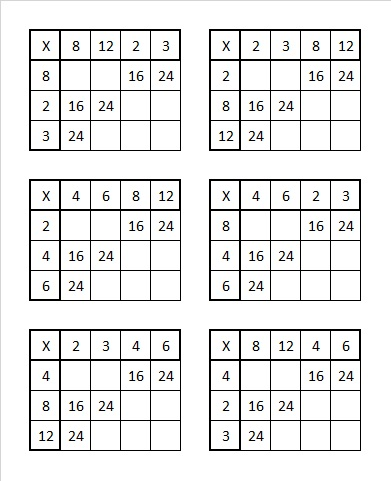# 1761 Irish Harp

Contents

### Today’s Puzzle:

This mystery-level puzzle was meant to look a little like an Irish harp. Using logic write the numbers 1 to 12 in the first column and again in the top row so that those numbers and the given clues make a multiplication table. There is only one solution.Notice that the clues 16 and 24 appear THREE times in the puzzle. In each case, you will need to determine if the common factor is 2, 4, or 8. You will have to get the common factor for each one in the right place or it will cause trouble for another clue. Consider what problems each of the following scenarios bring to other clues. For example, 48 must be either 4 × 12 or 6 × 8, but both possibilities are impossible in at least one of these scenarios:Once you determine the only scenario that doesn’t present a problem for any other clue, you will be able to begin the puzzle.

Here’s the same puzzle without any added color:### Factors of 1761:

1 + 7 + 6 + 1 = 15, a number divisible by 3, so 1761 is divisible by 3. Since 6 is divisible by 3, we didn’t have to include it in our sum: 1 + 7 + 1 = 9, so 1761 is divisible by 3.

• 1761 is a composite number.
• Prime factorization: 1761 = 3 × 587.
• 1761 has no exponents greater than 1 in its prime factorization, so √1761 cannot be simplified.
• The exponents in the prime factorization are 1 and 1. Adding one to each exponent and multiplying we get (1 + 1)(1 + 1) = 2 × 2 = 4. Therefore 1761 has exactly 4 factors.
• The factors of 1761 are outlined with their factor pair partners in the graphic below.### More About the Number 1761:

1761 is the difference of two squares in two different ways:
881² – 880² = 1761, and
295² – 292² = 1761.

1761 is palindrome 1N1 in base 32
because 1(32²) + 23(32) + 1(1) = 1761.

This site uses Akismet to reduce spam. Learn how your comment data is processed.